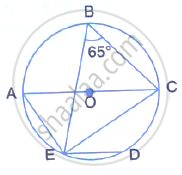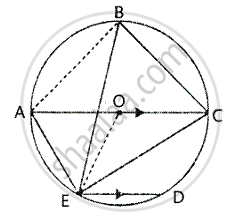Share

In the Given Figure, Chord Ed is Parallel to Diameter Ac of the Circle. Given ∠Cbe = 65°, Calculate ∠Dec. - ICSE Class 10 - Mathematics

ConceptArc and Chord Properties - the Angle that an Arc of a Circle Subtends at the Center is Double that Which It Subtends at Any Point on the Remaining Part of the Circle

Question

In the given figure, chord ED is parallel to diameter AC of the circle. Given ∠CBE = 65°, calculate ∠DEC.SolutionJoin OE.
Arc EC subtends ∠EOC at the centre and ∠EBC at the remaining Part of the circle.
∠EOC = 2 ∠EBC = 2 ×65° =130°

Now in ΔOEC, OE = OC      [radii of the same circle]

∴ ∠OEC = ∠OCE
But, in ΔEOC ,
∠OEC + ∠OCE + ∠EOC = 180° [Angles of a triangle]
⇒  ∠OCE + ∠OCE + ∠EOC = 180°
⇒ 2 ∠OCE + 130° = 180°
⇒ 2 ∠OCE = 180° -130°
⇒ 2 ∠OCE = 50°
 ∠OCE = (50°)/2 = 25°
∴ AC || ED          [Given]

∴ ∠DEC = ∠OCE
⇒ ∠DEC = 25°

Is there an error in this question or solution?

Video TutorialsVIEW ALL 

Solution In the Given Figure, Chord Ed is Parallel to Diameter Ac of the Circle. Given ∠Cbe = 65°, Calculate ∠Dec. Concept: Arc and Chord Properties - the Angle that an Arc of a Circle Subtends at the Center is Double that Which It Subtends at Any Point on the Remaining Part of the Circle.
S